# GSEB Class 7 Maths Notes Chapter 6 Triangles and Its Properties

This GSEB Class 7 Maths Notes Chapter 6 Triangles and Its Properties covers all the important topics and concepts as mentioned in the chapter.

## Triangles and Its Properties Class 7 GSEB Notes

Triangle and its parts:
Triangle: A triangle is a simple closed curve made up of three line segments. It is usually denoted by the greek letter delta (A). It has three vertices, three sides and three angles. In the following fig., we have ∆ABC. It has
1. Three sides namely: $$\overline{\mathrm{AB}}, \overline{\mathrm{BC}}, \overline{\mathrm{CA}}$$2. Three Angles namely: ∆BAC, ∆ABC and ∆BCA to be denoted by ∠A, ∠B, and ∠C respectively.

3. Three vertices A, B, C. The side opposite vertex A is BC. Angle opposite to side AB is AC, B is the vertex opposite to the side AC.

Interior Angles: In ∆ABC; ∆BAC, ∆ABC and ∆ACB are called the interior angles as they lies inside the triangle. In the figure shown alongside shaded angles are interior angles.(I) Classification according to sides:
(a) A scalene triangle – no two sides equal in length.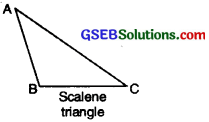(b) An isosceles triangle – two sides equal in length.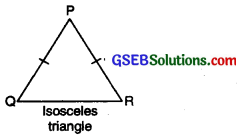(c) An equilateral triangle – all three sides equal in length.
Note: An equilateral triangle is also isosceles.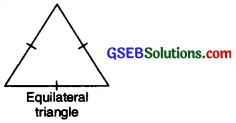(II) Classification according to angles:
(a) A right triangle or right-angled triangle – one angle is a right angle.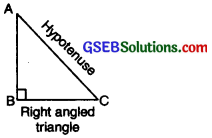(b) An obtuse-angled triangle – One angle is obtuse.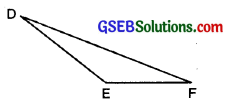obtuse-angled triangle
(c) An Acute-angled triangle – All angles are interior angles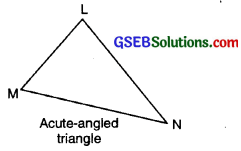Medians of a Triangle
A line segment that joins a vertex of a triangle to the mid-point of the opposite side is called a median of a triangle.

In the following fig., we have ∆ABC in which AD is the line segment drawn from the Vertex A to the mid-point D of the opposite side. This line segment AD is called the median from A to BC. Hence AD is the median of ∆ABC and it bisects the side BC into two halves where BD = DC. Similarly, BE and CF are other two medians: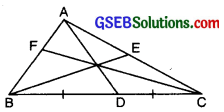Note:

• A triangle has three medians, all of which intersect at a point called ‘ ‘CENTROID’ ’.
• Medians lie completely in the interior of the triangle.
• A median divides the area of a triangle in two equal parts.
• The length of all the medians in an equilateral triangle is always equal.Altitudes of a Triangle
A line segment from a vertex of a triangle, Perpendicular to the line, segment containing the opposite side is called an altitude of the triangle.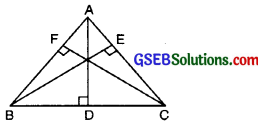In the above figure AD, BE and CF are the altitudes of ∆ABC drawn from the vertex A, B and C respectively.

Note:

• A triangle has three altitudes.
• The altitude is also called the height of the triangle.
• All the altitudes of an acute angled triangle lie inside the triangle.
• In an obtuse triangle, the altitude corresponding to the vertex with obtuse angle, lies inside the triangle, where as the other two altitudes lie outside the triangle.
• Two altitudes of a right angled triangle are actually the perpendicular legs of the triangle itself while the third lies inside the triangle.
• The equilateral triangle has all the altitudes of equal length.
• The altitudes of a triangle intersect at one point called “ORTHOCENTER”.

Exterior angle
An exterior angle of a triangle is formed, when a side of a triangle is produced. At each vertex, we have two ways of forming an exterior angle.

Exterior angle of a Triangle and its Property
If a side of a triangle is produced, the exterior angle so formed is equal to the sum of two interior opposite angles.
Proof: Let a sid BC of a ∆ABC be produced to D forming exterior ∠ACD.We know that sum of the angles of a triangle is 180°.
∴ ∠1 + ∠2 + ∠3 = 180°
But ∠3 + ∠4 = 180°
(By linear pair property)
∠1 + ∠2 + ∠3 = ∠3 + ∠4
⇒ ∠1 + ∠2 = ∠4For a given ∆ABC, if c B
side CA is produced to the point D. Then A is its exterior angle and if side BA is produced to the point E, then the exterior angle would be ∠CAE.

The two interior angles of a triangle opposite to the exterior angle are called its interior opposite angles. While the third opposite interior is called the adjacent interior angle.

In above triangle if ∠CAE is an exterior angle then ∠CAB is adjacent to ∠CAE. The remaining two angles ∠C and ∠B are called opposite interior angles.

Note: For a triangle, sum of an exterior angle and adjacent interior angle is always equal to 180° because every pair of exterior angle and its adjacent interior angle forms a linear pair.∴ For given ∆ABC.
∠BAC + ∠BAE = 180°
∠CBA + ∠CBF = 180°
∠ACB + ∠ACD = 180°

Angle Sum Property of a Triangle
Statement. The three angles of a triangle have a total measure of 180°.
To justify this let us use the exterior angle property of a triangle.Given ∠1, ∠2, ∠3 are angles of ∆ABC. 4 is the exterior angle when BC is extended to D.
Proof: ∠1 + ∠2 + ∠3 = ∠4 + ∠3 (by exterior angle property) = 180°
(as ∠4, ∠3 form a linear pair)
We can use this property in a number of ways.

Pythagoras Theorem
Right-angled Triangle and Pythagoras Property
Pythagoras, a Greek philosopher of sixth century BC (Before Christ), is said to have found a very important and useful property of right-angled triangles. The property is, hence named after him. Infact this property was known to people of many other countries too. The Indian mathematician Baudhayan has also given an equivalent form of this property.

Pythagoras property is a very useful tool in mathematics. It is formally proved as a theorem in later classes. You should be clear about its meaning.Pythagoras property states that for any right-angled triangle, the area of the square on the hypotenuse is equal to the sum of the areas of the squares on the legs.In given right angled triangle ;
The square of the hypotenuse
= Sum of the squares on the legs ⇒ a2 = b2 + c2

Conversely ; if Pythagoras property holds, the triangle must be right-angled.Triangle Inequality
Drawing of a triangle with given three sides is possible if sum of the lengths of any two sides of a triangle is greater than the third side. If a, b and c are three sides of a triangle, then
a + b > c
b + c > a
a + c > bIf any of three relations does not hold then no triangle is possible.